# Equipment and Instrumentation

27CHAPTER 2

Equipment and Instrumentation

Jonathan J. Halford

INTRODUCTION

The recognition of noncerebral signals in clinical neurophysiology (CNP) requires both the ability to recognize artifactual patterns and an understanding of the physics and engineering principles that are involved. Although most clinical neurophysiologists are adept at recognizing patterns, few have formal engineering or physics training. This should not discourage practitioners in the field of CNP, because most of the concepts relevant to clinical practice which underlie the technical aspect of their instruments can be supported using simple equations. The challenge is that the number of important concepts required to understand how the equipment works increases every year. Over the last several decades, the major driver of this evolution has been the digitization of recording. We all live in an analog world in which measured electrophysiologic values can take an infinite number of positive or negative values. Neurophysiological recordings began as a completely analog enterprise with equipment that channeled electrical currents which could take on an infinite set of values. Obviously, several decades ago this abruptly changed. Now all of the neurophysiology equipment on the market has a substantial digital component, meaning that the equipment operates using one or more internal computers that measures a limited number of possible discrete quantities.

As every year passes, we see more analog components of neurophysiology-recording equipment replaced by digital components. An example of this is the spread of digital equipment beyond the amplifier, storage, and visualization stages of the recording to the electrodes themselves in recent research using EEG recording systems. Another example is the change in how signals are filtered. Previously, this required only an understanding of analog electronics. Now, signal filtering is performed by both analog and digital equipment. The reason for this “digital spread” is that, although digital systems force the recorded signal to become discrete (potentially leading to a loss of relevant information), they are faster than analog systems, offer lower power consumption, allow greater control on signal information flow, and are less susceptible to noise.

Despite this digital spread, most artifacts in neurophysiology recordings have their source in the initial stages of the recording process, which are analog and will probably remain analog for a long time or indefinitely. For this reason, this chapter focuses on an explanation of analog recording concepts but also provides explanation of important digital recording concepts. Additionally, certain mathematical concepts in information theory will be presented when they are relevant to artifacts due to digital signal postprocessing and display.

ANALOG RECORDING

Basic Electrical Principles

The movement of electrical charge in currents within the nervous system leads to the electromyography (EMG), EEG, and magnetoencephalography (MEG) signal. Electric charges exist in two forms (with either positive or negative polarity) and are found in packages or “quanta” of a particular unit size—the negative charge of one electron or the positive charge of one proton. Protons are usually fixed within atoms and cannot move, but some electrons from each atom, especially in certain elements, are free to move 28between atoms. Their movement creates electrical current flow. Charges also create electrical fields which cause them to act at a distance upon other charges, with like charges repelling each other and opposite charges attracting. Electrical current flows best through materials called conductors, which are composed primarily of metals or solutions. When electric current flows through a conductor, it also creates a magnetic field around it. The measurement unit for charge (Q) is the coulomb, which carries the charge of 6.24 × 1018 electrons. Flow of electrical current (I) is measured in amperes, also called “amps,” and is equal to the flow of one coulomb of charge per second.Electrical current does not flow very fast, only approximately 0.25 mm/second, but the field of distribution spreads out within the conductor at the speed of light. This is similar to what happens in a pipe filled with water in which, when a small quantity of water is forced into one end of the pipe, the pressure increase within the water spreads very rapidly and a small quantity of water is immediately forced out of the other end of the pipe, despite the water itself in the pipe not having travelled far. Any changes to a magnetic field created by changes in the movement of electrical charge also spreads out from the conductor at the speed of light.

Electrical devices work by causing current to flow around in a circle, called a circuit. The voltage (V) is the amount of potential energy between two points in that circuit. The unit measure of V is the volt, which is the energy that is measured in joules which will be delivered per coulomb of charge when that charge passes between two points in the circuit. A common analogy to understand electric current flow is water flow to and from a water tank. The volume of water is equivalent to Q, the rate of flow is equivalent to I, and V is equivalent to the difference in the pressure of water between two points in the flow system (such as between the top of the water tank and the pipe in your home). Like an electrical circuit, water delivery in a city runs in a circle, with water flowing down from the tank to various destinations from which it is eventually recollected and pumped back into the tank. But unlike water, which usually flows only one way through pipes, electronic circuits are often designed to create current flow which alternates in direction very rapidly. A direct current (DC) is current that flows only in one direction. Alternating current (AC) rapidly switches direction of flow.

The electrical resistance (R) is a measure of the difficulty of passing an electrical current through a conductor. Generally, the amount of current (I) is linearly proportional to V and inversely linearly proportional to R:The unit of measure for R is ohm (Ω), which equals 1 volt per ampere of current. A resistor is an electrical circuit element added to create an impediment to current flow, like a sponge or filter placed in a pipe to slow the flow of water. A material with a high R, such as rubber, is called an insulator. Conductance (G) is defined as the opposite of resistance such that G = 1/R. The human body predominantly consists of salt water, which acts as a passive volume conductor, a relatively low-R medium for electric current through which an electric field can spread easily throughout. This is the reason why signals generated in one area of the body (such as the heart) can be measured in completely different areas of the body (such as on the head or foot).

Two circuit elements serve to store electric charge, capacitors, and inductors. Capacitors are devices used to store charge, consisting of one or more pairs of conductors (usually metal plates) separated by a thin insulator (Figure 2.1).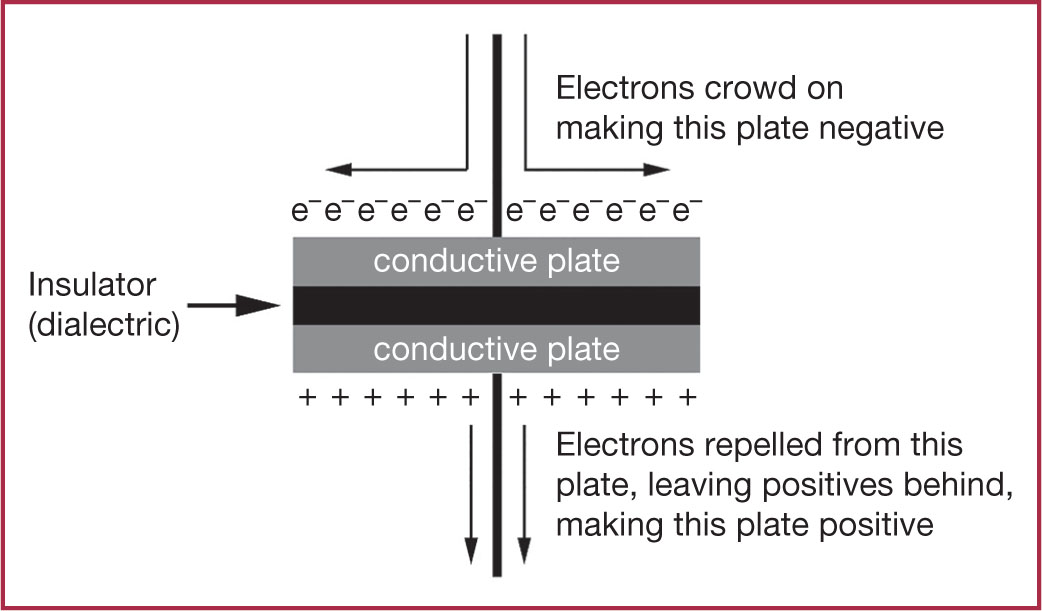FIGURE 2.1: Diagram of a capacitor.

A voltage source, such as a battery, pushes electrons (negative charge) onto one of the conductive plates, creating an electrical field that pushes electrons off the opposite plate, leaving positive charges behind. How much charge a capacitor can store, or its capacitance (C), is linearly proportional to the amount of charge stored in the capacitor (Q) and inversely linearly proportional to the voltage difference (V) between the two metal plate conductors.The unit of measurement of capacitance is the farad (F), which equals the capacitance in which one coulomb of charge causes a potential difference across the capacitor of one volt. A capacitor will resist the flow of DC but will allow AC 29to “flow” through, although this AC flow is more of an illusion since it is caused by electric charge accumulating or leaving the two plates of the capacitor. This apparent “flow” of current across a capacitor is termed capacitive coupling.

Inductors are electrical components that store energy in a magnetic field when electrical current is flowing through it. Typically they consist of a coil of wire wrapped around a magnet. When electrical current flows through an inductor, a magnetic field is created around it (Figure 2.2).

If the electrical current flowing through the inductor decreases or stops, the magnetic field quickly collapses and induces a current in the coil which resists that decrease in current flow. In this way, an inductor resists changes in the current, therefore resisting AC but permitting DC. The strength of an inductor to perform this function is termed inductance (L) and its unit of measurement is in henrys. In a circuit in which the current is decreasing at a constant rate of one ampere per second, an inductance of one henry results in the generation of one volt of potential difference across the inductor. This induced voltage, called the electromotive force, is directly proportional to L and is also directly proportional to the inverse of the change in current (dI).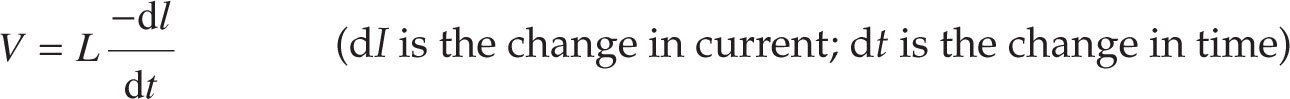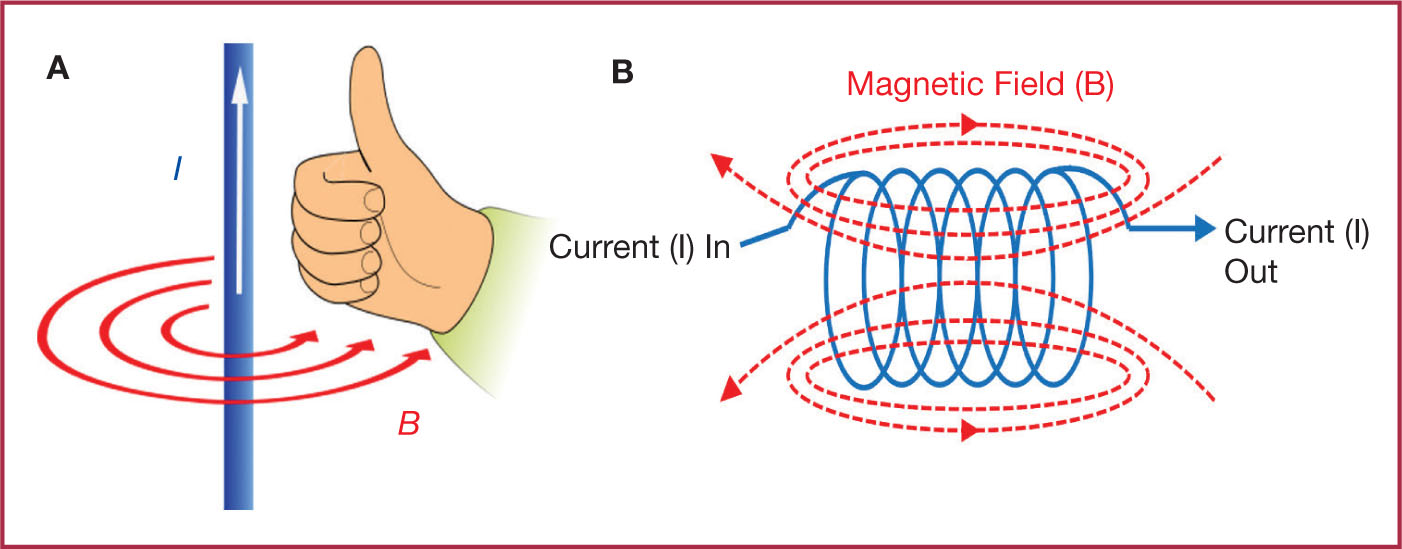FIGURE 2.2: (A) The right-hand rule illustrates the direction of the magnetic field if the electrical current is flowing upwards. (B) The magnetic field generated by electrical current flow through a coiled conductor.

Often there are multiple circuit elements that store charge in an electrical circuit and these sum in different ways, depending on whether they are in series or in parallel, as described in Table 2.1.30The fundamental circuit for understanding the analogue components of neurophysiology recording is the resistor–capacitor (RC) circuit (Figure 2.3). The current flow in this circuit varies over time when a voltage change occurs.FIGURE 2.3: (A) A series RC circuit is shown with three circuit elements: the resistor (R), capacitor (C), and a power source (Vin). Three voltage potential changes are labeled: the voltage potential increase from a power source (Vin), the voltage potential decrease across a resistor (VR), and the voltage potential decrease across a capacitor (VC). The current (I) flows clockwise around the circuit (1). (B) The current flow as a capacitor charges. Since the time constant for the RC circuit (τ) is 0.5 seconds, current drops to the 1/e (0.368) fraction of the starting current by that time point.

Analog Amplifiers

The changes in the current in an AC electrical signal are sinusoidal, meaning they follow the shape of a sine wave. Many biological signals have a sinusoidal pattern as well, and any time-varying signal can be broken down into a combination of sinusoidal waves of different frequencies and times of occurrence. One of the primary functions of neurophysiology instruments is to amplify a biological signal, which means to increase the power of the voltage and/or current of the time-varying biological signals. Neurophysiology amplifiers are predominantly voltage amplifiers and therefore the output of the amplifier is measured in volts. The gain of an amplifier (G) is the factor by which the amplifier increases the amplitude of the input signal. A voltage amplifier with a G of 2, for example, outputs a signal with double the voltage of the input signal.

Because amplifiers are constructed with resistors, capacitors, and inductors, they provide resistance to the flow of electrical current, which is dependent on frequency of the waves in the biological signal. (Frequency is a measurement of the number of sinusoidal waves passing per unit time and is measured in hertz, which is the number of cycles or waves per second.) The impedance of an amplifier is a measure of the overall resistance of the amplifier to current flow. It is quite complicated to calculate impedance because it involves multiple factors including the resistance of the resistors, capacitors, and inductors in the amplifier system, as well as the frequency of the waveforms that the amplifier is acting on. The impedance of an amplifier is important because, ideally, a recording device should not disturb the electrical current flow that it is trying to measure. If an amplifier has a high impedance, it will not allow much current to flow into it, and therefore not disturb the current it is trying to measure. This leads to a more accurate measurement of the voltage of the source. The following equation and subsequent Figure 2.4 depict the relationship of the impedance of the biological source and the measurement device (which includes an amplifier).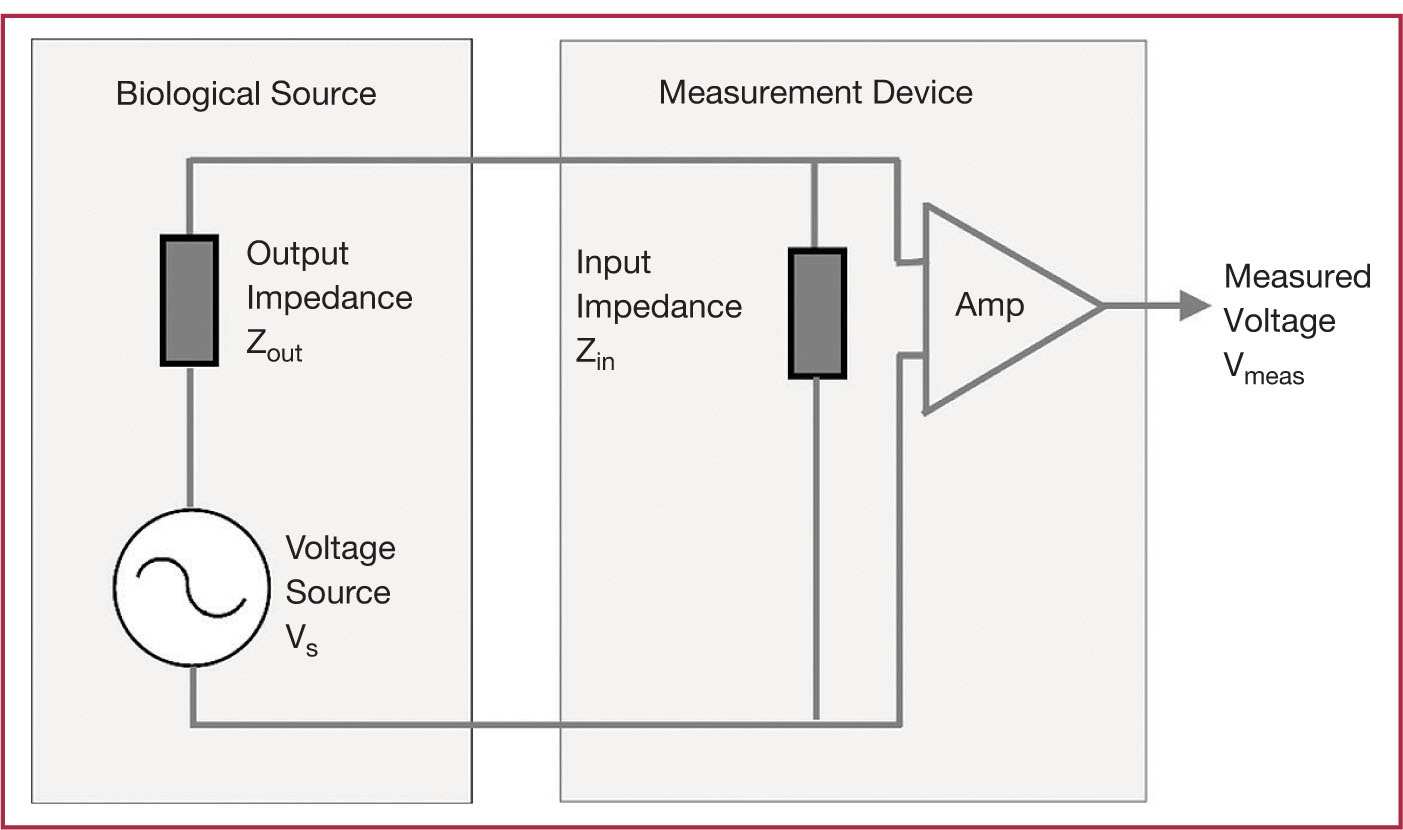FIGURE 2.4: Schematic of impedances and biological voltage source for a measurement device, which includes an amplifier.

31Note that if the Zin is much higher than the Zout, the impedance component of the equation is driven toward a value of one, causing the measured voltage to closely approximate the source voltage. If the value of Zin is near to the value of Zout, substantial current is shunted through the measurement device, disturbing the current flow through the biological source and decreasing the measured voltage.

As shown in Figure 2.4, an amplifier (also called an “amp”) has two input lines, which are usually wires, and it amplifies the voltage potential difference between these two lines to produce one output signal, which is called a channel. Each line is connected to an electrode placed on or in biological tissue. Usually one line is connected to a signal electrode, which is placed in a region of interest in the biological tissue, and one line is connected to a reference electrode, which is placed in a location with relatively low voltage. Biosignal recording equipment contains multiple amplifiers which permits recording from multiple signal electrodes. The reference electrode is connected to the second input of all of the amplifiers. This arrangement is called a referential recording. A ground electrode is also usually placed on the forehead in EEG recording. The location of the reference electrode in a referential EEG recording is usually chosen to be at the top of the head, at a distance remote from eye movement artifacts near the front of the head, muscle artifacts in the temporal regions, and the posterior dominant background rhythm in the posterior head region. Figure 2.5 contains a diagram of the wiring of an amplifier system in a referential recording setup with two signal electrodes, one referential electrode, and two amplifier output channels. This figure also shows the setup for bipolar recording, in which three electrodes are connected in a chain in which the middle electrode is connected to both amplifiers.

Because there is so much ambient noise in the environment during neurophysiologic recordings, a special type of amplifier, called a differential amplifier, is used in neurophysiology-recording equipment to minimize noise. This type of amplifier is essentially a combination of three amplifiers wired together. In the first stage of a differential amplifier, two amplifiers working in parallel amplify the potential difference between (a) the signal electrode and the patient ground electrode and (b) the reference electrode and the patient ground electrode. In the second stage, a third amplifier amplifies the difference between the outputs of the two first-stage amplifiers. A differential amplifier can be used for both a referential and a bipolar recording. Figure 2.6 depicts only the referential recording.

Environment noise usually causes a voltage potential difference between the recording electrodes and the ground electrode, which is called the common mode signal, and this is of relatively similar magnitude among the recording channels. The differential signals are the voltage potential differences between each signal electrode and the reference electrode, which are the signals of interest. Differential amplifiers work to cancel out much of the common mode signal, a process called common mode rejection. The degree to which a differential amplifier rejects the common mode signal is called the common mode rejection ratio (CMRR). This is calculated using the amplification of the common mode signal, called the common mode gain (Gcm) versus the amplification of the referential signal, called the referential gain (Gd). The CMMR is usually expressed in the unit of decibels (dB), which expresses the ratio of two numbers (usually signal magnitude) on a logarithmic scale multiplied by a factor of 20.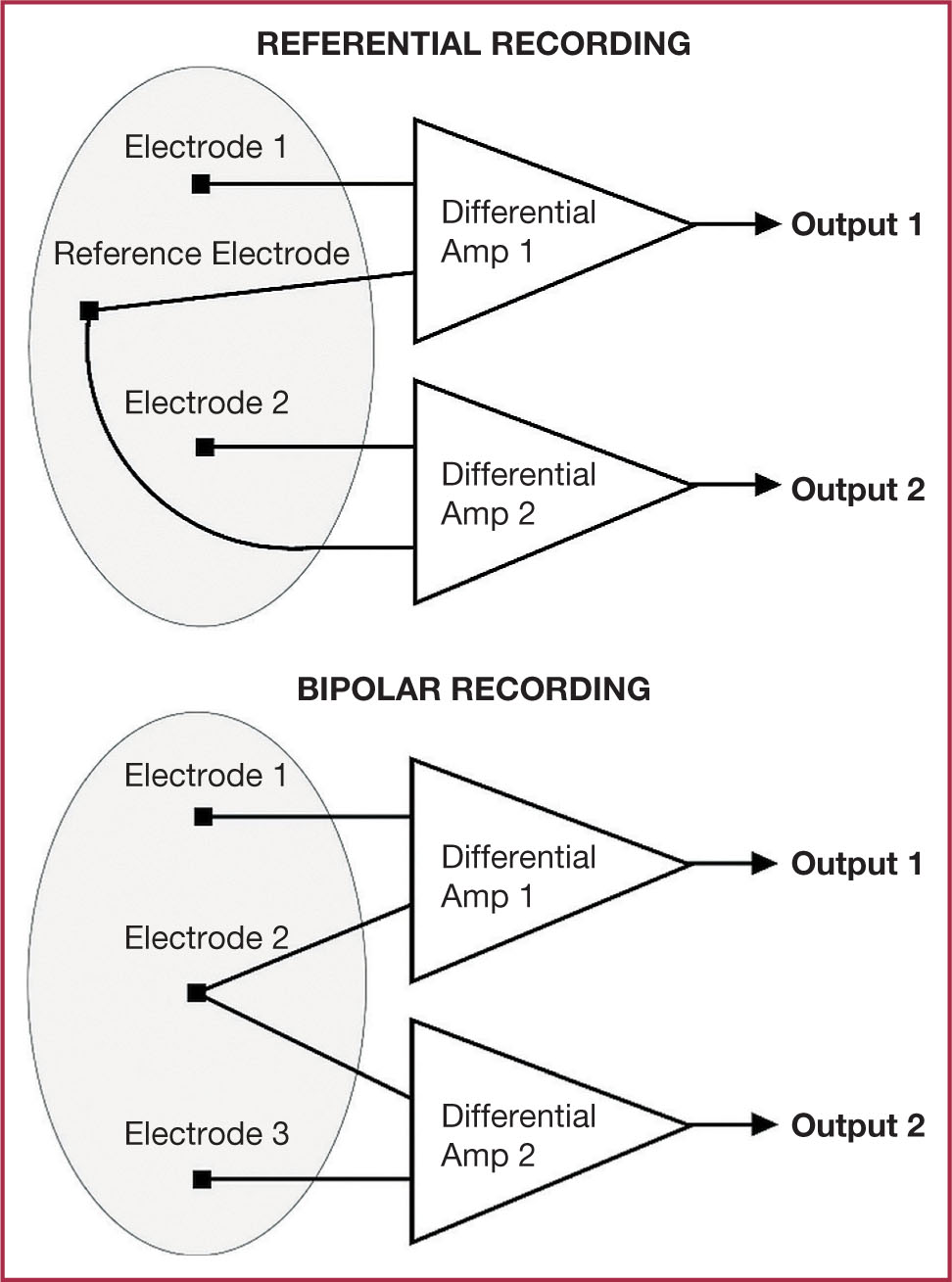FIGURE 2.5: Schematic depicting referential and bipolar recordings.